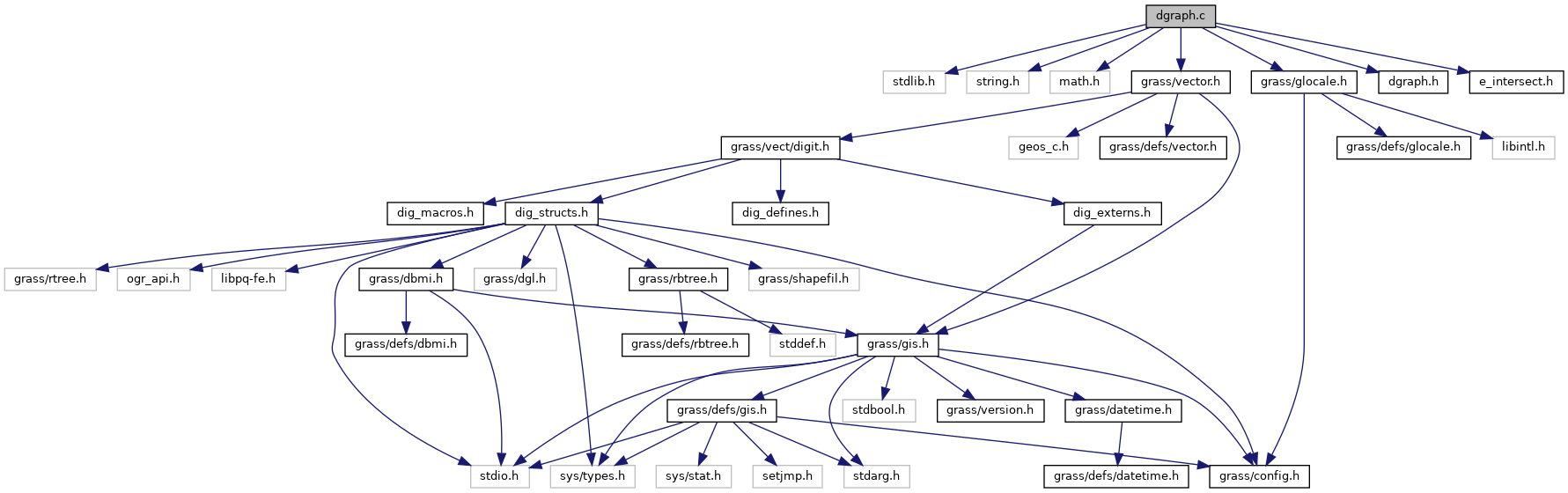GRASS GIS 7 Programmer's Manual  7.9.dev(2021)-e5379bbd7
dgraph.c File Reference

Vector library - intersection (lower level functions) More...

`#include <stdlib.h>`
`#include <string.h>`
`#include <math.h>`
`#include <grass/vector.h>`
`#include <grass/glocale.h>`
`#include "dgraph.h"`
`#include "e_intersect.h"`
Include dependency graph for dgraph.c:Go to the source code of this file.

## Macros

#define LENGTH(DX, DY)   (sqrt((DX*DX)+(DY*DY)))

#define MIN(X, Y)   ((X<Y)?X:Y)

#define MAX(X, Y)   ((X>Y)?X:Y)

#define PI   M_PI

## Functions

struct seg_intersections * create_si_struct (int segments_count)

void destroy_si_struct (struct seg_intersections *si)

void add_ipoint1 (struct seg_intersection_list *il, int with, double dist, int ip)

void add_ipoint (const struct line_pnts *Points, int first_seg, int second_seg, double x, double y, struct seg_intersections *si)

void sort_intersection_list (struct seg_intersection_list *il)

int compare (const void *a, const void *b)

double get_epsilon (struct line_pnts *Points)

struct seg_intersections * find_all_intersections (const struct line_pnts *Points)

struct planar_graphpg_create_struct (int n, int e)

void pg_destroy_struct (struct planar_graph *pg)

int pg_existsedge (struct planar_graph *pg, int v1, int v2)

void pg_addedge1 (struct pg_vertex *v, struct pg_edge *e)

void pg_addedge (struct planar_graph *pg, int v1, int v2)

struct planar_graphpg_create (const struct line_pnts *Points)

## Detailed Description

Vector library - intersection (lower level functions)

Higher level functions for reading/writing/manipulating vectors.

(C) 2008-2009 by the GRASS Development Team

This program is free software under the GNU General Public License (>=v2). Read the file COPYING that comes with GRASS for details.

Definition in file dgraph.c.

## ◆ LENGTH

 #define LENGTH ( DX, DY ) (sqrt((DX*DX)+(DY*DY)))

Definition at line 24 of file dgraph.c.

## ◆ MAX

 #define MAX ( X, Y ) ((X>Y)?X:Y)

Definition at line 29 of file dgraph.c.

Referenced by pg_create_struct().

## ◆ MIN

 #define MIN ( X, Y ) ((X

Definition at line 26 of file dgraph.c.

## ◆ PI

 #define PI   M_PI

Definition at line 31 of file dgraph.c.

## Function Documentation

 void add_ipoint ( const struct line_pnts * Points, int first_seg, int second_seg, double x, double y, struct seg_intersections * si )

Definition at line 120 of file dgraph.c.

 void add_ipoint1 ( struct seg_intersection_list * il, int with, double dist, int ip )

Definition at line 99 of file dgraph.c.

## ◆ compare()

 int compare ( const void * a, const void * b )

Definition at line 177 of file dgraph.c.

Referenced by MT_functions_new(), and rbtree_create().

## ◆ create_si_struct()

 struct seg_intersections* create_si_struct ( int segments_count )

Definition at line 64 of file dgraph.c.

References G_malloc, and NULL.

## ◆ destroy_si_struct()

 void destroy_si_struct ( struct seg_intersections * si )

Definition at line 85 of file dgraph.c.

References G_free().

## ◆ find_all_intersections()

 struct seg_intersections* find_all_intersections ( const struct line_pnts * Points )

Definition at line 218 of file dgraph.c.

## ◆ get_epsilon()

 double get_epsilon ( struct line_pnts * Points )

Definition at line 193 of file dgraph.c.

 void pg_addedge ( struct planar_graph * pg, int v1, int v2 )

Definition at line 416 of file dgraph.c.

 void pg_addedge1 ( struct pg_vertex * v, struct pg_edge * e )

Definition at line 405 of file dgraph.c.

References pg_vertex::eallocated, pg_vertex::ecount, pg_vertex::edges, and G_realloc.

## ◆ pg_create()

 struct planar_graph* pg_create ( const struct line_pnts * Points )

Definition at line 449 of file dgraph.c.

## ◆ pg_create_struct()

 struct planar_graph* pg_create_struct ( int n, int e )

Definition at line 352 of file dgraph.c.

## ◆ pg_destroy_struct()

 void pg_destroy_struct ( struct planar_graph * pg )

Definition at line 368 of file dgraph.c.

## ◆ pg_existsedge()

 int pg_existsedge ( struct planar_graph * pg, int v1, int v2 )

Definition at line 382 of file dgraph.c.

References pg_vertex::ecount, pg_vertex::edges, planar_graph::v, pg_edge::v1, and pg_edge::v2.